# Comparing different approaches to evolutionary simulations¶

## 4 Feb 2013¶

http://www.yoavram.com/

# Moran vs. Wright-Fisher¶

## Moran process¶

The simplest approach to model the change in frequency of alleles (i.e., evolution) is described by the Moran process, which can be explained as a type of a ball and urn game.

There is an urn with yellow blue balls. At each turn we draw two balls from the urn. The one in our right hand we replace by two balls of the same color, and the one in our left hand we discard.

Of course, in half of the cases the number of balls of each color doesn't change (when we draw two balls of the same color) and therefore we focus on cases in which we draw two different balls. The game can be rephrased as we draw a ball, replace it by two balls of the same color, and discard a ball of the opposite color.

The game ends when all balls are of the same color.

Denoting the number of yellow balls by x and the number of blue balls by y we can write a code for this game.

In :
def moran_draw(x, y):
r = randint(1, x + y + 1)
if r <= x:
return x + 1, y - 1
else:
return x - 1, y + 1

In :
def game(x, y, draw):
count = 0
while x != 0 and y != 0:
x, y = draw(x, y)
count += 1
return y == 0, count


We can run this game multiple times to check, for example, how the number of draws required to finish the game are distributed:

In :
def repeat(x, y, repeats, draw):
return [game(x, y, draw) for _ in range(repeats)]

In :
num_balls = [10, 50, 100, 200, 500, 800, 1000, 1250, 1400, 1500]

def run_and_summarize(draw, n_repeats=100):
results = array([repeat(n, n, n_repeats, draw) for n in num_balls])
means = results.mean(axis=1)
stds = results.std(axis=1)
return results, means, stds
moran_results, moran_means, moran_stds = run_and_summarize(moran_draw)

In :
plot_summary(num_balls, moran_means, moran_stds, game_name="Moran process")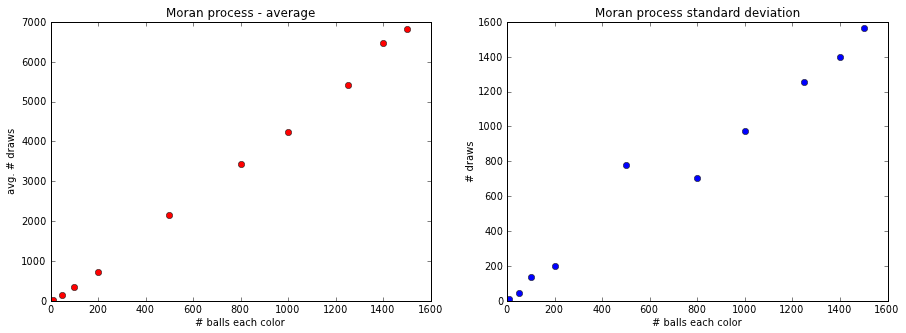In :
plot_histograms(moran_results, num_balls, game_name="Moran process")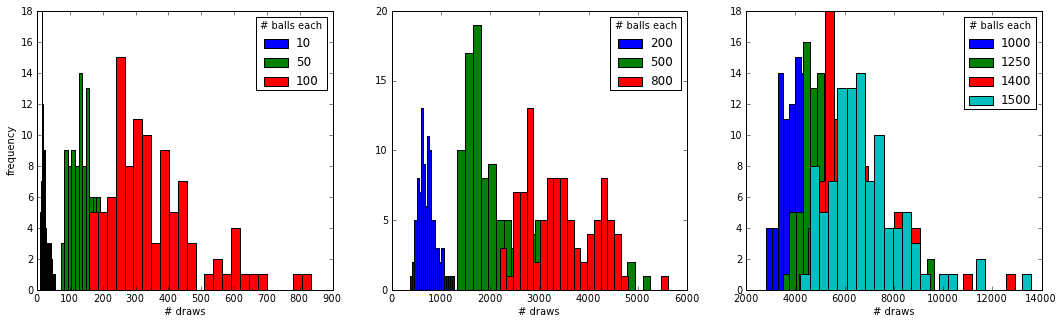### Markov Chain¶

A moran process can be described by a Markov chain. Denote the total number of balls by $n$ and use this probability matrix (for n=2):

in which $A_{i,j}$ is the probablity to transition from j yellow balls to i yellow balls where there is a total of n=2 balls in the game.

### Evolutionary model¶

From an evolutionary perspective, this setup models genetic drift, but it can be expanded is much the same way to include mutation and selection (although adding selection will disrupt the linearity of the above model), and even recombination in a multi-locous model.

## Wright-Fisher process¶

In the Wirght-Fisher (WF) process, we replace the entire contents of the urn at each step of the game, drawing the next generation of balls from the current urn with replacement.

At first, this sounds the same as the Moran process if we zoom out and look at a generation as n draws, where n is the total number of balls. But in effect the processes are different, because in the WF process the draws within the generation are independent, but in the Moran process they are not. This leads to stronger drift and faster extinctions in the Moran process, as we will soon see.

The WF process can be modeled by using a binomial random function (or a multinomial, if there are multiple types/loci):

In :
def wf_draw(x, y):
n = x + y
x = binomial(n, x / float(n))
y = n - x
return x, y


Note that we only changed the drawing function, but now a draw is a whole generation, whereas in the Moran process it was a single reproduction and death step, so that a generation was regarded as n draws, where n is the total number of balls.

In :
wf_results, wf_means, wf_stds = run_and_summarize(wf_draw)
plot_summary(num_balls, wf_means, wf_stds, "Wright-Fisher process")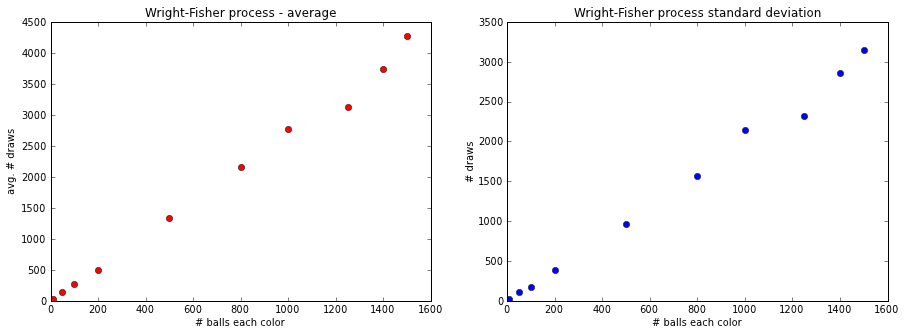Note that the time scales (on the y-axis) are different from those of the Moran process because in the WF process the entire population is replaced on each tick, whereas in the Moran process a single individual is replaced on each tick.

The fact that the sacles, although different, are on the same order of magnitude suggests that the time for extinction of an allele in the Moran process is much shorter, as it requires several thousands of reproductions instead of several thousands of generations. This is a result of the fact that in the Moran process the sampling is without replacement whereas in the WF process the sampling is with replacement.

In :
plot_histograms(wf_results, num_balls)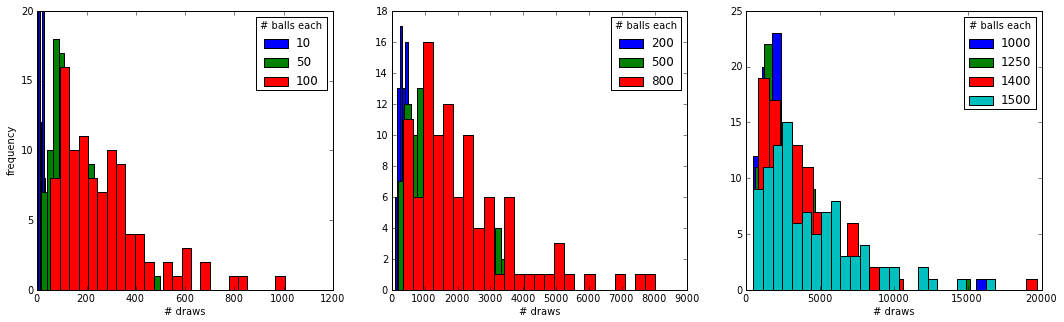## Computational comparison¶

In the WF model we use one random function call per generation, whereas in the Moran process we use n calls, where n is the total number of balls. On the other hand, the Moran process tends to finish in a smaller number of generations because drift is stronger. I therefore compare the running time of both processes for a small population and a large one:

In :
%timeit game(100, 100, moran_draw)
%timeit game(100, 100, wf_draw)

1000 loops, best of 3: 365 us per loop
1000 loops, best of 3: 458 us per loop


In :
%timeit -n 100 game(10**6, 10**6, moran_draw)
%timeit -n 100 game(10**6, 10**6, wf_draw)

100 loops, best of 3: 8.4 s per loop
100 loops, best of 3: 4.56 s per loop



For the small population the Moran process was slightly faster. For the large population, the WF model was slightly faster.

So if I were to require 25,000 games to produce a good dataset for a research project, the Moran process would take

In :
print "%.2f seconds for a small population" % (25000*365.0*10**-6)
print "%.2f days for a large population" % (25000*8.4/(24*60*60))

9.12 seconds for a small population
2.43 days for a large population



and the WF process would take

In :
print "%.2f seconds for a small population" % (25000*458.0*10**-6)
print "%.2f days for a large population" % (25000*4.56/(24*60*60))

11.45 seconds for a small population
1.32 days for a large population



# Individual-based vs. Density-based simulation¶

## Individual-based simulation¶

In individual-based simulations (also called agent-based simulations) all the attributes of every individual, such as fitness, genome and modifiers, are explicitly modeled.

To illustrate an example of an individual-based simulation, the following code implements a Wright-Fisher process with drift, selection and mutation. The genome is represented by the number of mutations accumulated, and all mutations are deleterious with a constant effect on fitness.

We start from a mutation-free constant size population and proceed for a pre-defined number of generations, en route to a mutation-selection balance in which the mean number of deleterious mutations in the population is stable around $\mu/s$, where $\mu$ is the mutation rate and $s$ is the selection coefficient.

In :
class Organism:
def __init__(self, mutations, mutation_rate, selection_cofficient):
self.mutations = mutations
self.mutation_rate = mutation_rate
self.selection_cofficient = selection_cofficient
self.fitness = self._fitness()

def reproduce(self):
child = Organism(self.mutations, self.mutation_rate, self.selection_cofficient)
child.mutations += poisson(self.mutation_rate)
child.fitness = self._fitness()
return child

def _fitness(self):
return (1 - self.selection_cofficient) ** self.mutations

def __repr__(self):
return "*"+str(self.mutations)

In :
import random

def selection_mutation_IBS(population):
fitness = array([o.fitness for o in population])
fitness = fitness/fitness.sum()
count = multinomial(len(population), fitness)
i = 0
for o,n in zip(population, count):
while n > 0:
population[i] = o.reproduce()
n -= 1
i += 1
return population

def drift_IBS(population):
return random.sample(population, len(population))

In :
def mutation_selection_balance_IBS(ticks, population_size, mutation_rate, selection_coefficient):
population = [Organism(0, mutation_rate, selection_coefficient) for i in range(population_size)]
for t in range(ticks):
population = drift_IBS(population)
population = selection_mutation_IBS(population)
mutation_load[t] = mean([o.mutations for o in population])

In []:
generations, population_size, mu, s = 100, 10**5, 0.003, 0.1
population_IBS, mutation_load_IBS = mutation_selection_balance_IBS(generations, population_size, mu, s)

In :
plot_mutation_load(mutation_load_IBS, mu, s, "Individual-based simulation")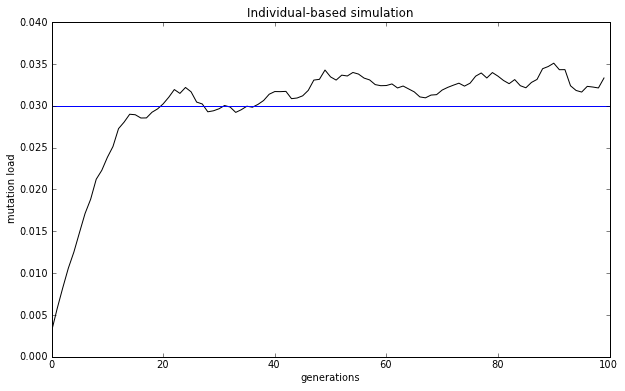## Density-based simulation¶

In a density-based simulation (and also in frequency-based simulations, which are only slightly different) the population is divided into types or classes of individuals that share the same set of attributes (alleles, modifiers, etc.). Then, these types are explicitly modeled, and the individuals are only implicitly modeled via the types they belong to.

This framework is very efficient because it allows the use of general algorithms, such as mutlinomial random number sampling.

Here is the code for a density-based simulation based on the same assumptions as the individual-based simulation above:

In :
def selection_DBS(population, population_size, selection_coefficient):
fitness = array([(1 - selection_coefficient) ** i for i in range(len(population))]) * population
fitness = fitness/fitness.sum()
return multinomial(population_size, fitness)

def drift_DBS(population, population_size):
population = population/float(population.sum())
return multinomial(population_size, population)

def mutation_DBS(population, mutation_rate):
mutations = poisson(mutation_rate * population)
population[:-1] = population[:-1] - mutations[:-1]
population[1:] = population[1:] + mutations[:-1]
return population

In :
def mutation_selection_balance_DBS(ticks, population_size, max_num_mutations, mutation_rate, selection_coefficient):
population = array( * max_num_mutations)
population = population_size
for t in range(ticks):
population = drift_DBS(population, population_size)
population = selection_DBS(population, population_size, selection_coefficient)
population = mutation_DBS(population, mutation_rate)
mutation_load[t] = sum([i * x for i,x in enumerate(population) if x > 0])/float(population.sum())

In []:
population_DBS, mutation_load_DBS = mutation_selection_balance_DBS(generations, population_size, 100, mu, s)

In :
plot_mutation_load(mutation_load_DBS, mu, s, "Density-based simulation")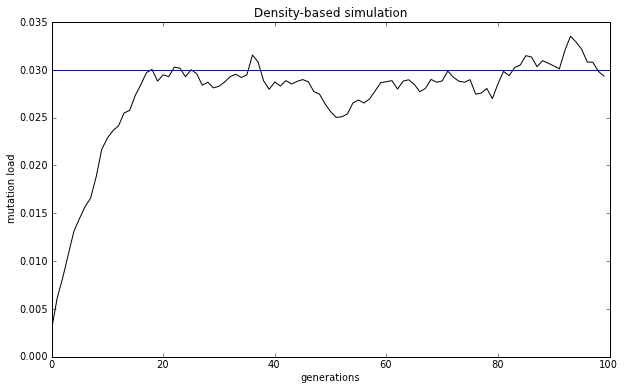## Computational comparison¶

### Running time¶

In individual-based simulations, because each individual is explicitly modeled, it is easier to write and test the simulation code, but it will likely be slow, because at every generation the complexity is O(n), as the simulation code must process each and every individual.

In a density-based simulations every type/class of individuals is explicitly modeled, and therefore the code is expected to run much faster, as long as the number of types is smaller than the number of individuals. This is expected to occur when the mutation and recombination rates are low and the population is large (i.e., in asexual populations). This gain in performance is paid by a more complex code which requires a minimal understanding of probability theory.

The below performance comparison shows that the density-based simulation is ~3800 times faster!

In :
%timeit mutation_selection_balance_IBS(10, population_size, mu, s)
%timeit mutation_selection_balance_DBS(10, population_size, 100, mu, s)

1 loops, best of 3: 14.6 s per loop
100 loops, best of 3: 3.78 ms per loop



### Memory use¶

If the population size is, say, $10^5$ individuals, and each has 1,000 loci in his genome, then the population will occupy ~100 megabytes of memory. If the population size increases to $10^7$, then the population will occupy 10 gigabytes of memory, which is already not feasible on most machines and not efficient when it is feasible.

The following prints the memory footpring of the individual-based and density-based populations:

In :
import sys
print "Individual-based population: %d bytes" % sys.getsizeof(population_IBS)
print "Density-based population: %d bytes" % sys.getsizeof(population_DBS)

Individual-based population: 800064 bytes
Density-based population: 80 bytes



The individual-based population is 10,000 times larger than the density-based population.

# Fin¶

This presentation was written using the IPython notebook.

The code for this presentation is available on GitHub.

The presentation can be viewed online on slideviewer, or you could install the latest versions of IPython (0.14.dev) and nbconvert - follow this recipe.

The presentation is published under a a Creative Commons Attribution-NonCommercial-ShareAlike 3.0 Unported License.In []: